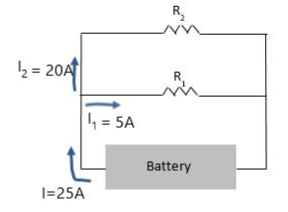# Problem: The current I in the main branch of the circuit shown is 25 Amps. The current I1 through resistor R1 is 5 Amps, and the current I2 through resistor R2 is 20 Amps. Does R1 or R2 have a higher value of resistance? a. R1 is larger than R2 b. R2 is larger than R1 c. There is not enough information

###### FREE Expert Solution

Ohm's law:

$\overline{){\mathbit{i}}{\mathbf{=}}\frac{\mathbf{V}}{\mathbf{R}}}$

Solving for resistance:

$\overline{){\mathbf{R}}{\mathbf{=}}\frac{\mathbf{V}}{\mathbf{i}}}$, where V is the voltage and i is the current.

100% (91 ratings)###### Problem Details

The current I in the main branch of the circuit shown is 25 Amps. The current I1 through resistor R1 is 5 Amps, and the current I2 through resistor R2 is 20 Amps. Does R1 or R2 have a higher value of resistance?a. R1 is larger than R2

b. R2 is larger than R1

c. There is not enough information

Frequently Asked Questions

What scientific concept do you need to know in order to solve this problem?

Our tutors have indicated that to solve this problem you will need to apply the Resistors and Ohm's Law concept. You can view video lessons to learn Resistors and Ohm's Law. Or if you need more Resistors and Ohm's Law practice, you can also practice Resistors and Ohm's Law practice problems.